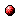# Linear model predictive control based on approximate optimal control inputs and constraint tightening

Reference:
I. Necoara, V. Nedelcu, T. Keviczky, M.D. Doan, and B. De Schutter, "Linear model predictive control based on approximate optimal control inputs and constraint tightening," Proceedings of the 52nd IEEE Conference on Decision and Control, Florence, Italy, pp. 7728-7733, Dec. 2013.

Abstract:
In this paper we propose a model predictive control scheme for discrete-time linear time-invariant systems based on inexact numerical optimization algorithms. We assume that the solution of the associated quadratic program produced by some numerical algorithm is possibly neither optimal nor feasible, but the algorithm is able to provide estimates on primal suboptimality and primal feasibility violation. By tightening the complicating constraints we can ensure the primal feasibility of the approximate solutions generated by the algorithm. Finally, we derive a control strategy that has the following properties: the constraints on the states and inputs are satisfied, asymptotic stability of the closed-loop system is guaranteed, and the number of iterations needed for a desired level of suboptimality can be determined.Corresponding technical report: pdf file (190 KB)
Note: More information on the pdf file format mentioned above can be found here.

Bibtex entry:

@inproceedings{NecNed:13-038,
author={I. Necoara and V. Nedelcu and T. Keviczky and M.D. Doan and B. {D}e Schutter},
title={Linear model predictive control based on approximate optimal control inputs and constraint tightening},
booktitle={Proceedings of the 52nd IEEE Conference on Decision and Control},